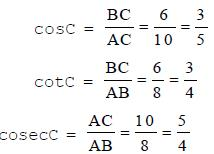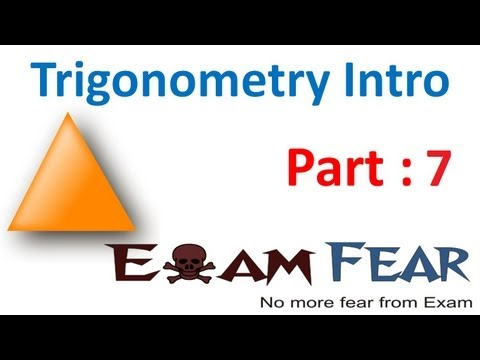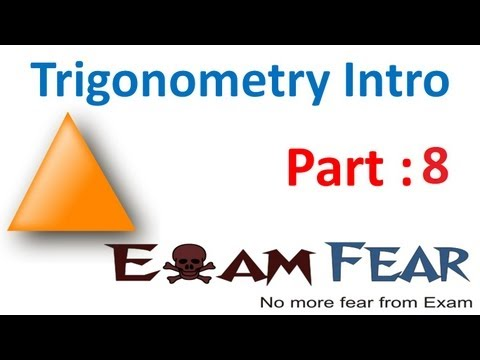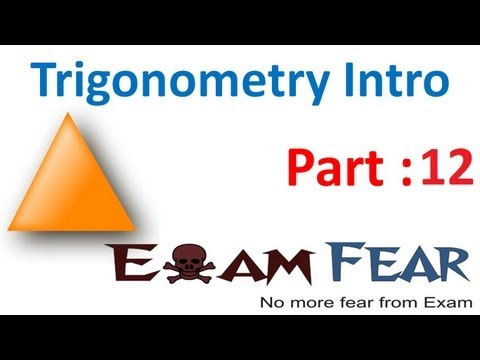Courses

# Trigonometric Ratios - Introduction To Trigonometry, Class 10 Mathematics

## 25 Questions MCQ Test | Trigonometric Ratios - Introduction To Trigonometry, Class 10 Mathematics

Description
This mock test of Trigonometric Ratios - Introduction To Trigonometry, Class 10 Mathematics for Class 10 helps you for every Class 10 entrance exam. This contains 25 Multiple Choice Questions for Class 10 Trigonometric Ratios - Introduction To Trigonometry, Class 10 Mathematics (mcq) to study with solutions a complete question bank. The solved questions answers in this Trigonometric Ratios - Introduction To Trigonometry, Class 10 Mathematics quiz give you a good mix of easy questions and tough questions. Class 10 students definitely take this Trigonometric Ratios - Introduction To Trigonometry, Class 10 Mathematics exercise for a better result in the exam. You can find other Trigonometric Ratios - Introduction To Trigonometry, Class 10 Mathematics extra questions, long questions & short questions for Class 10 on EduRev as well by searching above.
QUESTION: 1

Solution:
QUESTION: 2

Solution:
QUESTION: 3

### When the image is of the same size as the object, the magnification is

Solution:
QUESTION: 4

The name and radius of curvature of a spherical mirror whose focal length is +2 cm is​

Solution:
QUESTION: 5

No refraction occurs when light goes from​

Solution:
QUESTION: 6

An object is situated at a distance of f/2 from a convex lens of focal length f. Distance of image will be​

Solution:
QUESTION: 7

After reflection through a concave mirror, rays of light

Solution:
QUESTION: 8

Virtual image forms

Solution:
QUESTION: 9

Which lens always forms a virtual image?

Solution:
QUESTION: 10

What will happen when a ray enters from glass to air medium?

Solution:
QUESTION: 11

If the magnification has a negative sign, the image formed by the concave mirror must be

Solution:
QUESTION: 12

An object 4 cm tall is placed in front of a convex lens. It produces an image 3 cm tall. What is the magnification of the lens?​

Solution:
QUESTION: 13

Two thin lenses of power +3.5 D and -2.5 D are placed in contact. Find the focal length of the lens combination.​

Solution:
QUESTION: 14

Rahul is standing in front of a plane mirror. The distance between Rahul and his image is 150cm. If Rahul’s distance from the mirror is x. What could be the possible value of x?​

Solution:
QUESTION: 15

The mirror used as rear view mirror is​

Solution:
QUESTION: 16

Which one of the following materials cannot be used to make lens?

Solution:
QUESTION: 17

In a convex lens, where is the image formed, when an object is placed at 2F?​

Solution:
QUESTION: 18

If the radius of curvature of a spherical mirror is 34.52 cm, then the focal length of that spherical mirror is​

Solution:
QUESTION: 19

The focal length of a plane mirror is

Solution:
QUESTION: 20

A ray of light passes through the focus of the mirror. After reflection, it

Solution:
QUESTION: 21

An object is placed on the principal axis of a spherical mirror. The object distance u is:

Solution:
QUESTION: 22

The mirror formula is valid for​

Solution:
QUESTION: 23

Raj is standing in front of a plane mirror. The distance between Raj and his image is 100cm. If the distance between the mirror and image is x. What could be the possible value of x?​

Solution:
QUESTION: 24

The ratio of sine of angle of incidence to the sine of angle of refraction equals refractive index is called​

Solution:
QUESTION: 25

When the image is of double size as the object, the magnification is

Solution:

Track your progress, build streaks, highlight & save important lessons and more!

### Similar Content### Related tests